EE - 456 / E01 spring 2003

Professor: Arida

Submitted By:

Andrew Buettner

Lab #1: Quantization

Sunday, March 02, 2003

1) Cover Page 1

3) Objective 3

4) Components Used 3

5) Procedures 3

6) Lab Data / Results 3

1) Table 1 3

2) Diagram 1 4

3) Diagram 2 4

4) Diagram 3 5

5) Diagram 4 5

6) Diagram 5 6

7) Diagram 6 6

8) Diagram 7 7

7 Answers to Lab Questions 7

7) Conclusions 8

8) Attachments 8Objective

The objective of this lab is to quantize sinewaves and compute the signal-to-noise ratio. The results will be compared to a predicted value that will be computed through a given formula.Components Used

1) Computer with Octave installedProcedures

1) Generate a 512 sample sinewave with f=1Hz and an amplitude of 1023

2) Plot the signal

3) Quantize the signal using the command "xq = 128*round(x./128);"

4) Plot both functions

5) Create function "d" that is the difference between "x" and "xq"

6) Plot all three functions.

7) Calculate the variance using "vx=(x*x')./511"

8) Calculate the variance of d using a similar method

9) Calculate the distortion ratio using SDR=vx/vd

10) Compare to an expected distortion ratio 6*(A/q)^2

11 Repeat steps 3 - 9 for quantization of 5, 6, 7, and 8 bits, only plot the culmination of all three functions.Lab Data / Results

1) Table 1: Signal to Distortion Results

Number of Bits

Quantization Interval

Observed SDR

Expected SDR

% Error

Q = 4

128

415.96

383.3

8.52%

Q = 5

64

1622.1

1553.0

4.45%

Q = 6

32

6418.7

6132.0

4.68%

Q = 7

16

24974

24528

1.82%

Q = 8

8

97060

98112

1.07%

2) Diagram 1: Original Sinewave3) Diagram 2: Original Sinewave and 4 bit Quantized Wave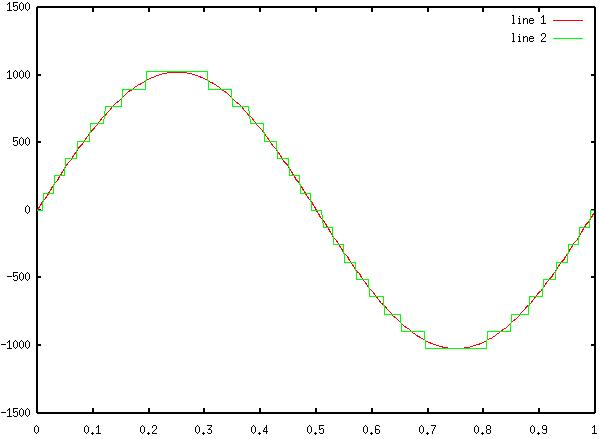4) Diagram 3: Original Sinewave, 4 bit Quantized, and Difference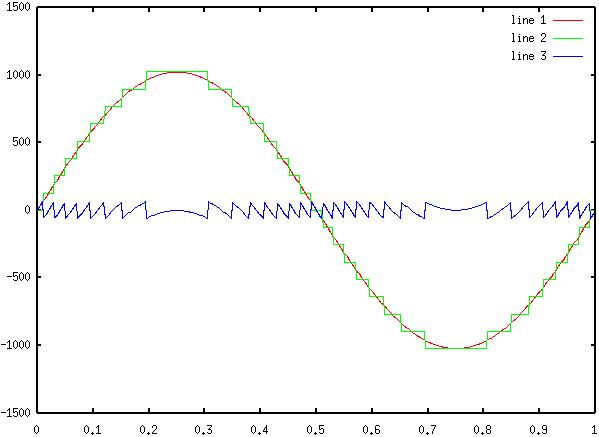5) Diagram 4: Original Sinewave, 5 bit Quantized, and Difference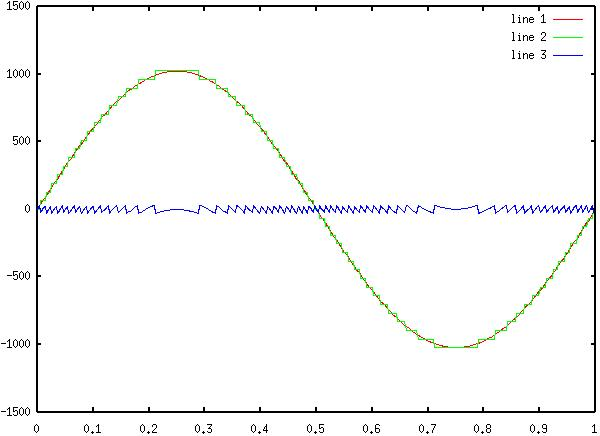6) Diagram 5: Original Sinewave, 6 bit Quantized, and Difference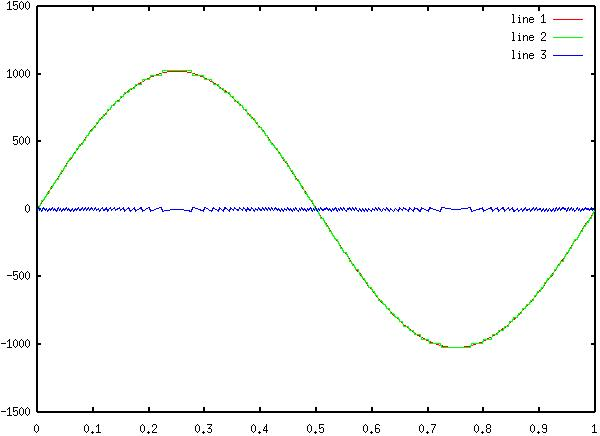7) Diagram 6: Original Sinewave, 7 bit Quantized, and Difference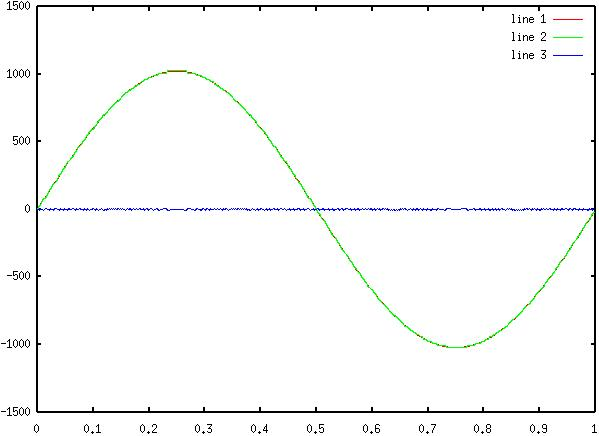8) Diagram 7: Original Sinewave, 8 bit Quantized, and Difference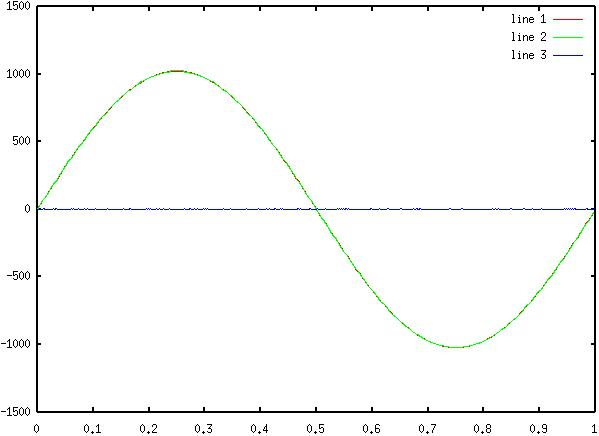Answer to Lab Questions

1) Q: Derive the formula SDR=6*(A/q)2

A: See Attachments::Calculations

2) Q: Given a quantized array, derive a set of MatLab® commands that will discover the number of bits used to encode the aray.

A: Assuming the signal is sinusoidal and has an absolute average of 0

1) vxq=(xq*xq')./N; (N is the number of samples in xq)

2) int=max(xq) - min(xq);

3) q=round(log(int)/log(2))

3) Q: What formula would be used to describe the signal - to - distortion ratio if truncation were used, instead of rounding.

A: SDRT Z 3*(A/q)2Conclusions

This lab has demonstrated the effect of voltage quantizing on a signal. It has illustrated two different methods of computing the signal - to distortion ratio. Both methods yield fairly accurate results (<10% error). It is interesting that the higher the number of bits used in quantization, the lower the error was in predicting the SDR. This gives a practical formula (S 1% for eight or more bits) for quantization. However, I believe that the ratio of deviations is a much more accurate formula, and actually yielded the correct results, as opposed to the formula. It appears that the value 6 needs to be greater for larger quantization interval, and less for smaller intervals.Attachments

1. Original lab handout

2. Original lab data

3. Calculations

4. Octave log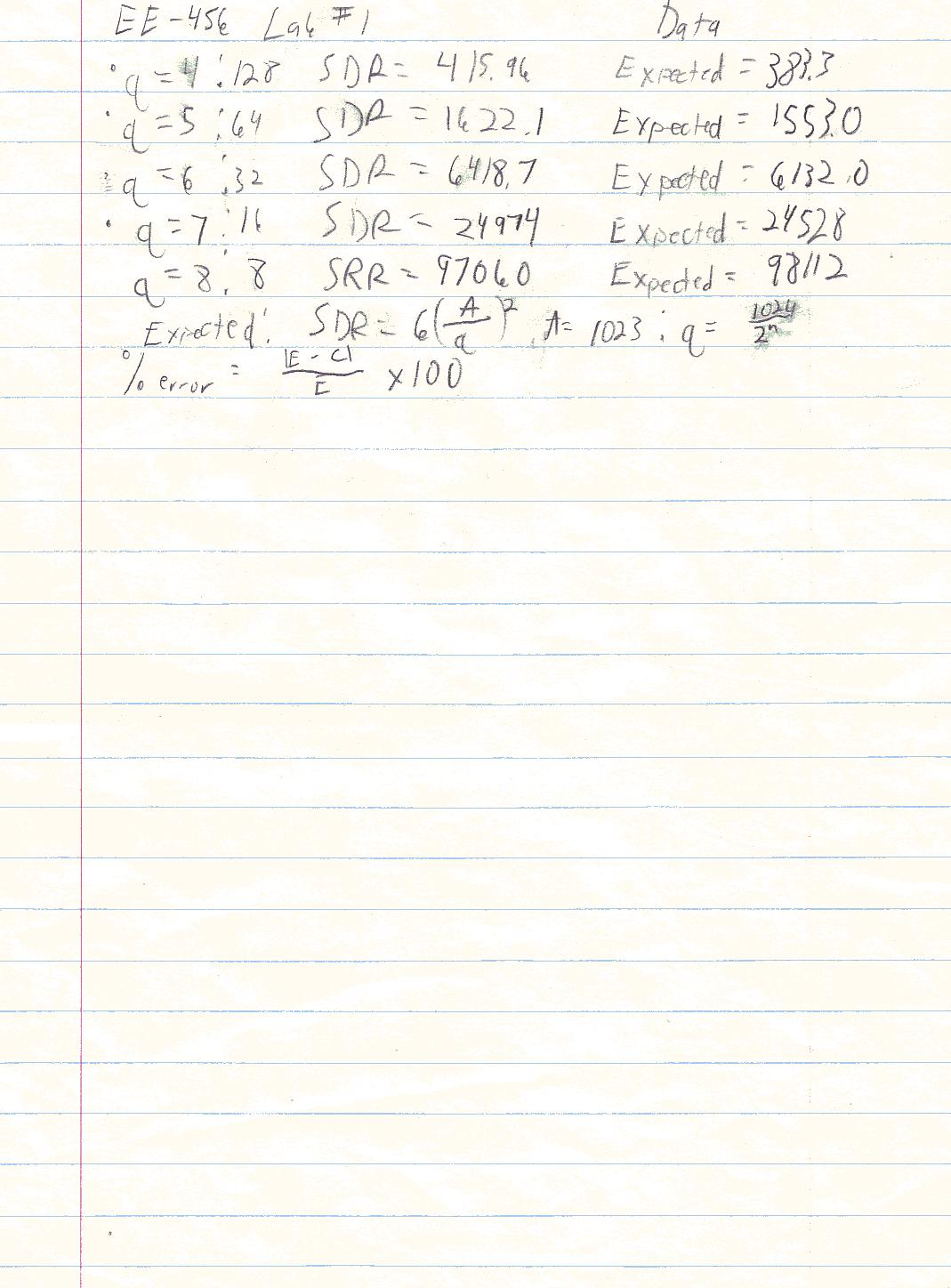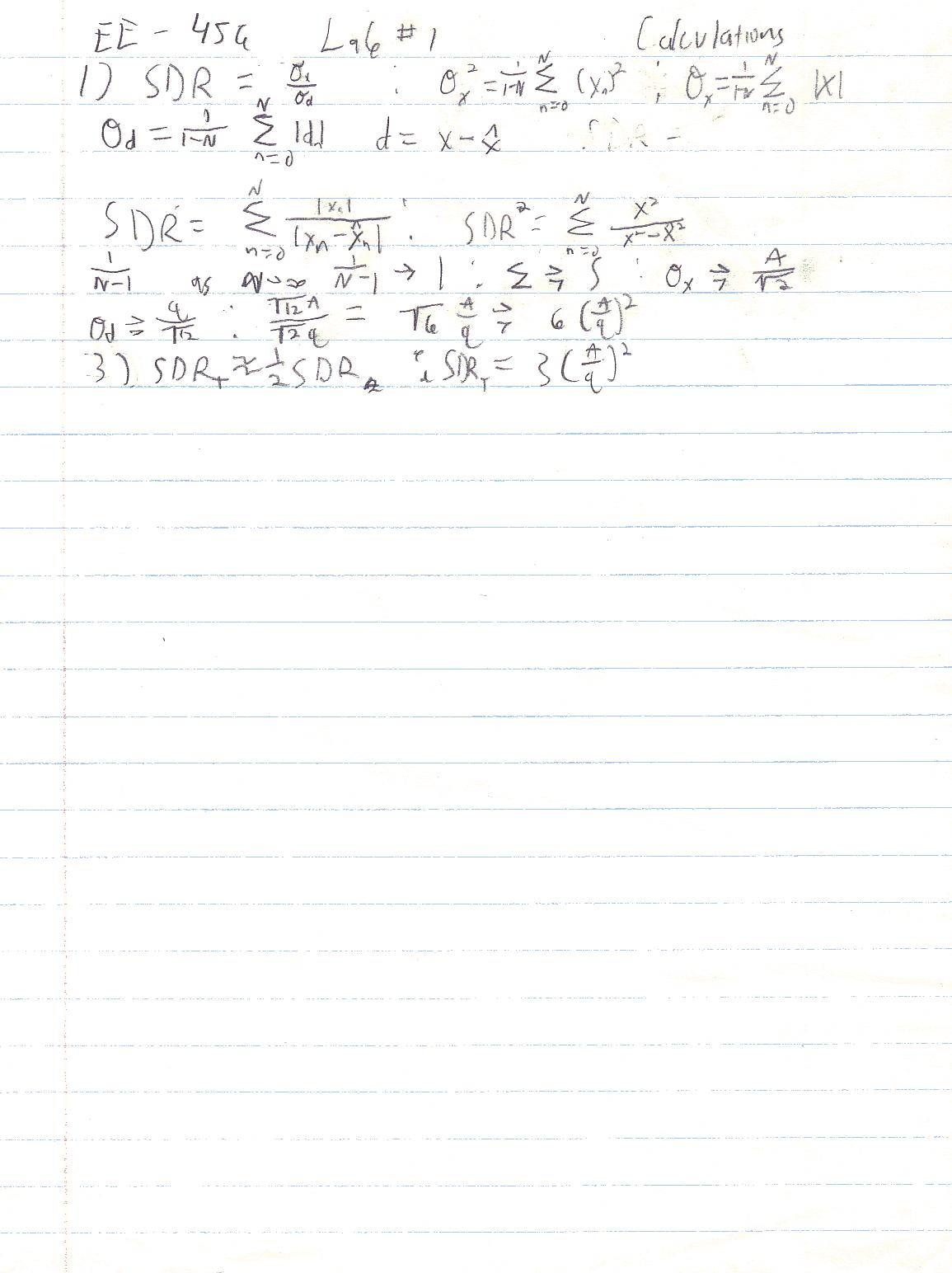GNU Octave, version 2.0.17 (i686-pc-linux-gnu).

Copyright (C) 1996, 1997, 1998, 1999, 2000, 2001, 2002 John W. Eaton.

This is free software with ABSOLUTELY NO WARRANTY.

For details, type `warranty'.

octave:1> n=0:511;

octave:2> t=n/512;

octave:3> x=1023*sin(2*pi*t);

octave:4> plot(t,x)

octave:5> xq=128*round(x./128);

octave:6> plot(t,x,t,xq)

octave:7> d=x-xq;

octave:8> plot(t,x,t,xq,t,d)

octave:9> vx=(x*x')./512

vx = 5.2326e+05

octave:10> vx=(x*x')./512;

octave:11> vd=(d*d')./511;

octave:12> vx=(x*x')./511;

octave:13> SDR=vx/vd

SDR = 415.96

octave:14> 1023/128

ans = 7.9922

octave:15> 2047/128

ans = 15.992

octave:16> 2048/(2^5);

octave:17> 2048/(2^5)

ans = 64

octave:18> xq5=64*round(x./64);

octave:19> d5=x-xq5;

octave:20> plot(t,x,t,xq5,t,d5)

octave:21> xd5=(d5*d5')./511;

octave:22> SDR5=vx/vd5

error: `vd5' undefined near line 22 column 9

error: evaluating expression near line 22, column 9

error: evaluating binary operator `/' near line 22, column 8

error: evaluating assignment expression near line 22, column 5

octave:22> vd5=(d5*d5')./511;

octave:23> SDR5=vx/vd5

SDR5 = 1622.1

octave:24> 2048/(2^6);

octave:25> 2048/(2^6)

ans = 32

octave:26>

octave:26> xq6=32*round(x./32);

octave:27> d6=x-xq6;

octave:28> plot(t,x,t,xq6,t,d6)

octave:29> vd5=(d5*d5')./511;

octave:30> vd6=(d6*d6')./511;

octave:31> SDR6=vx/vd6

SDR6 = 6418.7

octave:32> 2048/(2^7)

ans = 16

octave:33> xq7=16*round(x./16);

octave:34> d7=x-xq7;

octave:35> plot(t,x,t,xq7,t,d7)

octave:36> vd7=(d7*d7')./511;

octave:37> SDR=vx/vd7

SDR = 2.4974e+04

octave:38> 2048/(2^8)

ans = 8

octave:39> xq8=8*round(x./8);

octave:40> d8=x-xq8;

octave:41> plot(t,x,t,xq8,t,d8)

octave:42> vd8=(d8*d8')./511;

octave:43> SDR8=vx/vd8

SDR8 = 9.7060e+04

octave:44> plot(t,x,t,xq7,t,d7)

octave:45> vxq=(xq5*xq5')./511

vxq = 5.2749e+05

octave:46> log(xvq)/log(2)

error: `xvq' undefined near line 46 column 5

error: evaluating expression near line 46, column 5

error: evaluating argument list element number 1

error: evaluating index expression near line 46, column 1

error: evaluating binary operator `/' near line 46, column 9

error: evaluating assignment expression near line 46, column 9

octave:46> max(xq8)

ans = 1024

octave:47> min(xq8)

ans = -1024

octave:48> xvq/2048

error: `xvq' undefined near line 48 column 1

error: evaluating expression near line 48, column 1

error: evaluating binary operator `/' near line 48, column 4

error: evaluating assignment expression near line 48, column 4

octave:48> vxq/2048

ans = 257.57

octave:49> int=vxq/2048

int = 257.57

octave:50> q

error: `q' undefined near line 50 column 1

error: evaluating expression near line 50, column 1

octave:50> q=log(int)/log(2)

q = 8.0088

octave:51> round(q)

ans = 8

octave:52>